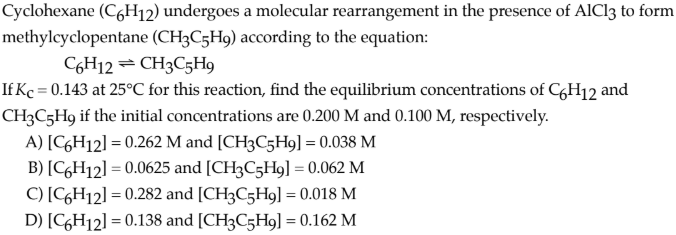# Cyclohexane (C6H12) undergoes a molecular rearrangement in the presence of AlCl3 to form methylcyclopentane (CH3C5H9) according to the equation: C6H12 ⇌ CH3C5H9 IfKc = 0.143 at 25°C for this reaction, find the equilibrium concentrations of C6H12 and CH3C5H9 if the initial concentrations are 0.200 M and 0.100 M, respectively. A) [C6H12] = 0.262 M and [CH3C5H9] = 0.038 M B) [C6H12] = 0.0625 and [CH3C5H9] = 0.062 M C) [C6H12] = 0.282 and [CH3C5H9] = 0.018 M D) [C6H12] = 0.138 and [CH3C5H9] = 0.162 M